• Slides: 15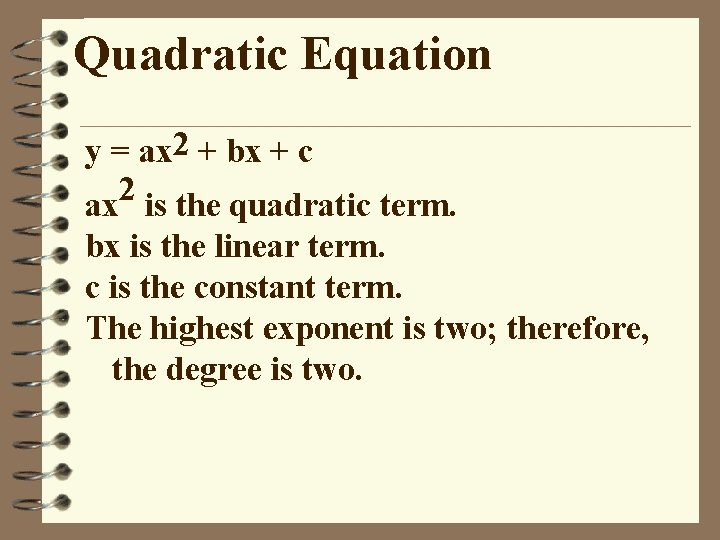Quadratic Equation y = ax 2 + bx + c 2 ax is the quadratic term. bx is the linear term. c is the constant term. The highest exponent is two; therefore, the degree is two.Identifying Terms 2 Example f(x)=5 x -7 x+1 Quadratic term 5 x 2 Linear term -7 x Constant term 1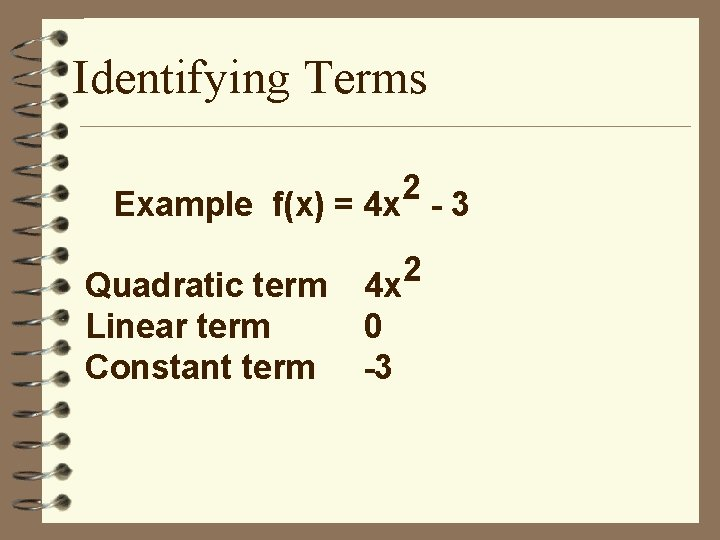Identifying Terms 2 Example f(x) = 4 x - 3 Quadratic term Linear term Constant term 4 x 0 -3 2Identifying Terms Now you try this problem. 2 f(x) = 5 x - 2 x + 3 quadratic term linear term constant term 5 x 2 -2 x 3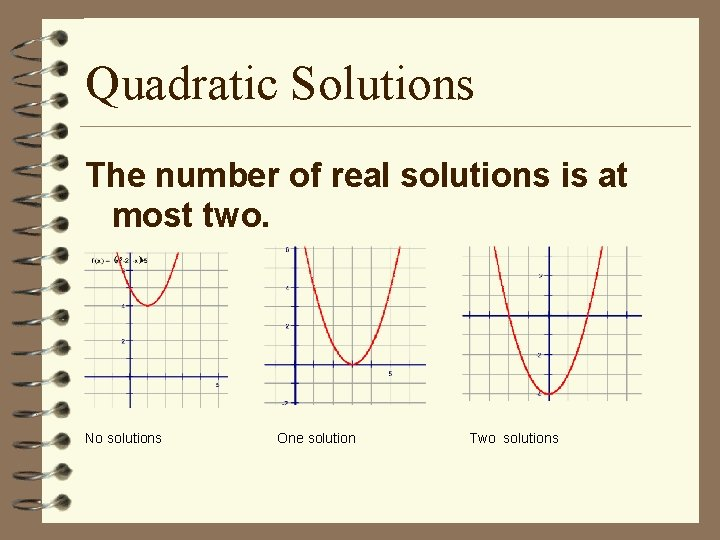Quadratic Solutions The number of real solutions is at most two. No solutions One solution Two solutions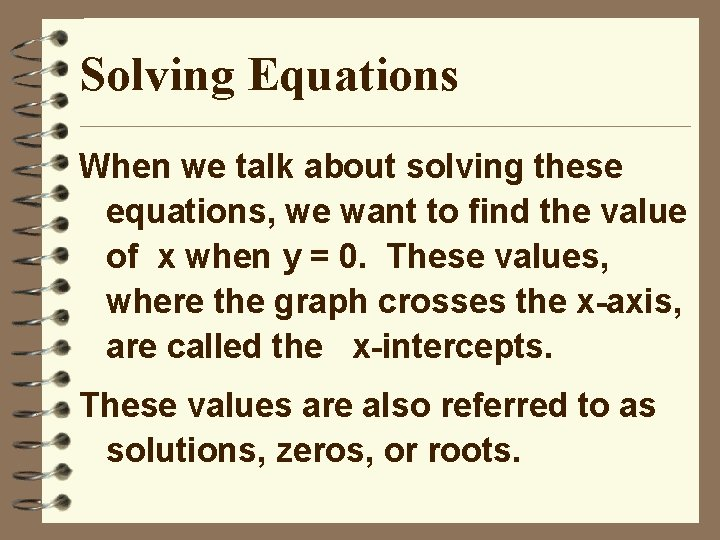Solving Equations When we talk about solving these equations, we want to find the value of x when y = 0. These values, where the graph crosses the x-axis, are called the x-intercepts. These values are also referred to as solutions, zeros, or roots.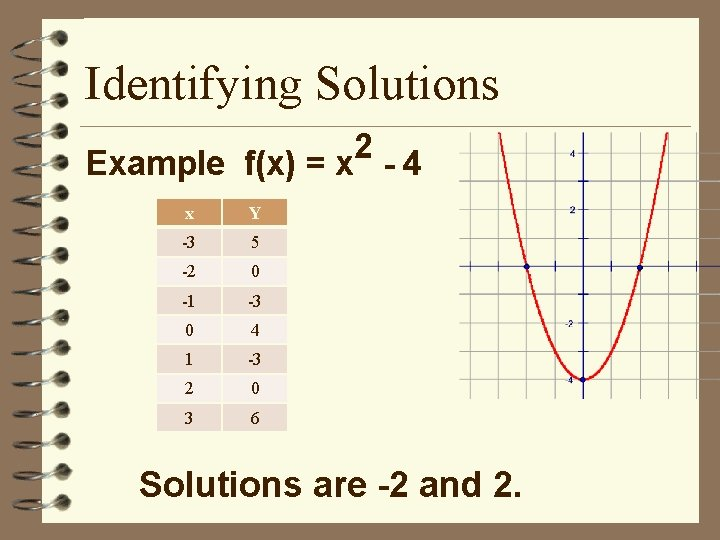Identifying Solutions 2 Example f(x) = x - 4 x Y -3 5 -2 0 -1 -3 0 4 1 -3 2 0 3 6 Solutions are -2 and 2.Identifying Solutions Now you try this problem. f(x) = 2 x 2 x X Y -2 -8 -1 -3 0 0 1 1 2 0 Solutions are 0 and 2.Graphing Quadratic Equations Graph y = x 2 - 4 x x y 0 1 2 3 4 0 -3 -4 -3 0 Roots 0 and 4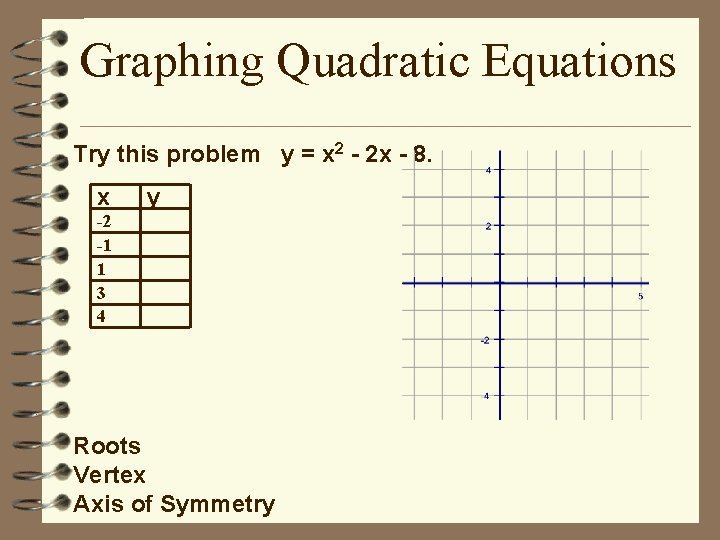Graphing Quadratic Equations Try this problem y = x 2 - 2 x - 8. x y -2 -1 1 3 4 Roots Vertex Axis of Symmetry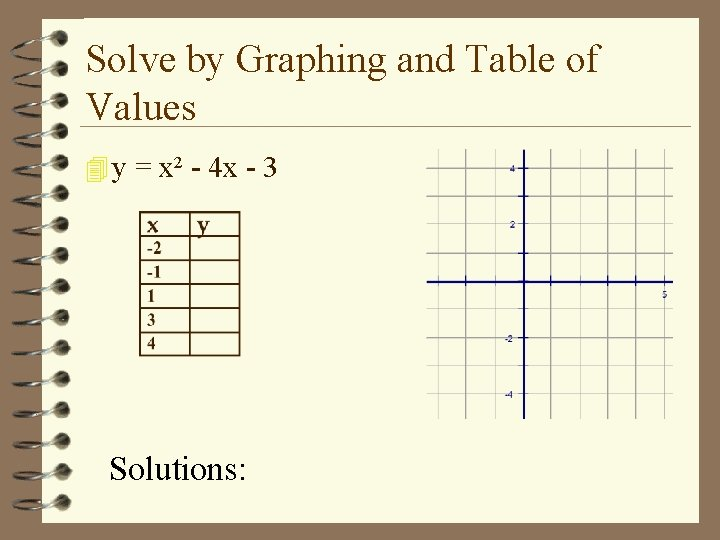Solve by Graphing and Table of Values 4 y = x² - 4 x - 3 Solutions: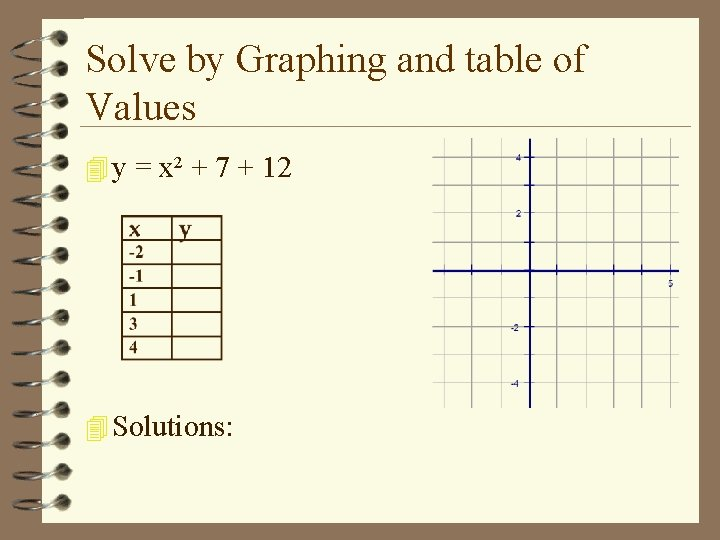Solve by Graphing and table of Values 4 y = x² + 7 + 12 4 Solutions:Factor and solve for x x² + 8 x + 7=0 Factor for a = 1 Set each set of parenthesis equal to 0 (x + 1) (x + 7) x+1=0 x+7=0 Solve for x 1 step x = -1 x = -7 Undo addition/subtractionYou Try 1. x² - 3 x – 4 = 0 x=4 x = -1 2. x² + 38 x + 72 = 0 x = -36 x = -2 3. x² + 15 x + 36 = 0 x = -12 x = -3